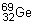Describe the property of radioactive decay, Chemistry

Assignment Help:

Q. Describe the Half -Life  property of radioactive decay?

Ans.

The decay of a nucleus is a random event. It is impossible to predict which nucleus will decay and when. Over a period of time, though, the decay rate is constant which allows us to predict when a given fraction of the sample has decayed.

The half-life is the amount of time it takes for half of a chunk of radioactive isotope to undergo radioactive decay. It is usually denoted by the symbol t1/2.

The half-life of germanium-69 is 36 hours. If we start with n atoms of, then at the end of 36 hours we will have n/2 (half) of the atoms ofleft. The rest of the atoms will have changed into another element or isotope. At the end of the next 36 hours (that's 72 hours from the starting point) there will be n/4 atoms ofleft. After another 36 hours have passed (108 hours from the beginning) there will be n/8atoms. See the trend? After each half-life we have half of the previous amount.

Calculate the number of photons per second, What is the percentage of light...

What is the percentage of light that is engaged by the sample after a path length of 1 µm? Calculate the number of photons per second absorbed by the sample.   T

Hardness, define hardness and its type?

define hardness and its type?

Rancidification - chemical properties of oils and fats, Rancidification - c...

Rancidification - chemical properties of oils and fats On long storage in contact along with air and moisture, oils and fats develop unlikable smell. The process is termed as r

Determine the crude fibre content in the given of flour, Q. Determine the c...

Q. Determine the crude fibre content in the given of flour? To determine the crude fibre content in the given sample of flour. After undertaking this activity, you will be a

Conductors, what is conductors?

what is conductors?

Engneering level, formation of scales

formation of scales

Rusting of iron, How rusting of iron is envisaged as setting up of an elect...

How rusting of iron is envisaged as setting up of an electrochemical cell? Solution) Rusting of Iron is due to corossion. Rust is an Fe 2 O 3 .xH 2 O Theory of Rusting :

Arhenious concept, Explain Arhenious theory with utility and limitation?

Explain Arhenious theory with utility and limitation?

The total number of orbitals in an energy level designated, The total numbe...

The total number of orbitals in an energy level designated by principal quantum number  n is equal to: (1) 2n                    (2) 2n 2            (3) n

IUPAC NAMES, how to iupac name

how to iupac name